Next: Conclusion, Part I Up: number11 Previous: The controller equations

We will start with the Laplace transform of the plant equation (5), since its a little simpler to do. The first step is to use the linearity property of Laplace transforms, which is given as the third rule in Table 2. This property allows us to do the transform of (5) by doing the transform of each term separately,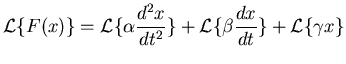(6)

and further the linearity property allows us to move the constants outside of the transform operation,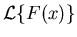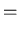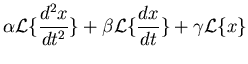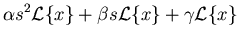(7)

Now representing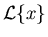as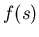, we get,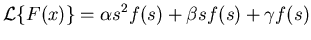(8)

The transfer function is the ratio of the output responseto the input forcing, which we get by rearranging the above equation to get,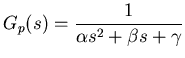(9)

Now that we see how we go about doing this, its a straightforward matter to do the same for the PID controller,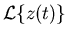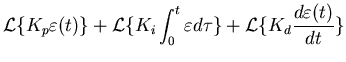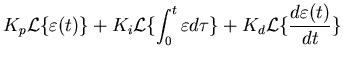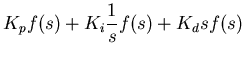(10)

(we needed the derivative rule, number 4, and the integral rule, number 7, from Table 2 as well as the linearity rule). Now we have to be careful here, the transfer function is the ratio of the output to the input. For the PID controller the error signal,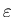, is the input, the output is the quantity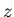.

So the transfer function is for the PID controller is,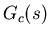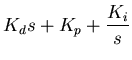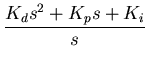(11)

Now we need to couple the controller and the plant. We will connect the output of the controller into the plant by taking the controller output, multiplying it by a plant gain factor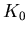, and using that as the plant input. This is represented mathematically by multiplying the controller response by the plant gain and the plant response,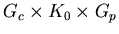We will connect the plant output into the controller at the negative side of the summing node (to get an error signal) after multiplying it by a feedback gain factor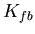. The plant and feedback gains do not really change anything, they just give us more opportunities to adjust things. The real change is the fact that the input to the controller is now reinterpreted as the output of the summing point (before is was just the input'', now we care how it relates to the rest of the system). So now part of the system input is the system output,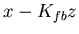We get the full equation by going step at a time through the diagram (figure 1). At the output of the summing node we have,then after the controller we get,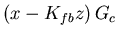and so after the plant we have,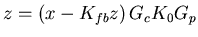(12)

The response function is the ratio of the outputto the input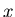which we can determine by reorganizing the above equation to get,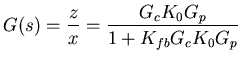(13)

now we expand this using (9) for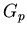and (11) for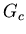and simplify,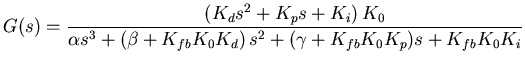(14)

This is the response function of our PID controller with the plant defined by equation (5). Notice that we managed to go from a description of how the controller is interconnected (basically Figure 1) all the way to its input response function with nothing more than polynomial manipulations. If we had not used Laplace tranforms, getting the response function by the direct manipulations of the integro-differential equation would have been much harder to do.

It is important to recognize that the details of what we have done are dramatically dependent upon the forms of equations (4) and (5). However the method that we used will apply as long as the two equations (and how they are coupled) are linear.

Now that we know how the adaptive controller will respond, how do we adapt it ? First we need to understand what it means for the controller to be optimally adapted. To do this, we need to consider when the denominator of equation (14) is zero.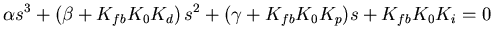(15)

This equation is called the characteristic equation for the system. For the moment we will combine the coefficients,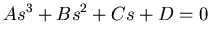(16)

The locations of the solutions to (16) in the complex plane can be used to predict the behaviour of the controller. For a given set of coefficients, there will be three solutions called the roots. If the roots are in the negative half of the plane, then the controller will be stable. If the roots are real but unequal, then the system is overdamped (that is, it will never quite recover from a suddenly imposed step input). If the roots are imaginary, then the system is underdamped (which will ring'' when it gets a step input). The optimal response to an imposed step is the critically damped case, this will happen if the roots are real and equal.

So our goal is to adjust the gains (the various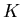values) so that the characteristic equation always has real, equal, negative roots. It turns out that we can achieve all these constraints provided that in equation (16),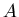and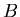have opposite signs and that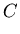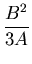(17)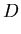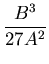(18)

Clearly, we cannot optimally adaptively control an arbitrary plant. If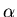and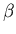don't have opposite signs then the controller won't even be stable. Also note that there are not enough equations to give us independently all five gains. The feedback and plant gainsandare either going to have to be defined to have fixed (known) values or they will need to be absorbed into the other gains.Next: Conclusion, Part I Up: number11 Previous: The controller equations
Skip Carter 2008-08-20